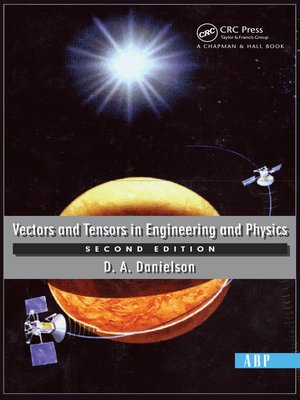# Vectors and Tensors In Engineering and Physics

#### byDonald Danielson

ebook

With an OverDrive account, you can save your favorite libraries for at-a-glance information about availability. Find out more about OverDrive accounts.

### Subjects

Vectors and Tensors in Engineering and Physics develops the calculus of tensor fields and uses this mathematics to model the physical world. This new edition includes expanded derivations and solutions, and new applications. The book provides equations for predicting: the rotations of gyroscopes and other axisymmetric solids, derived from Euler's equations for the motion of rigid bodies; the temperature decays in quenched forgings, derived from the heat equation; the deformed shapes of twisted rods and bent beams, derived from the Navier equations of elasticity; the flow fields in cylindrical pipes, derived from the Navier-Stokes equations of fluid mechanics; the trajectories of celestial objects, derived from both Newton's and Einstein's theories of gravitation; the electromagnetic fields of stationary and moving charged particles, derived from Maxwell's equations; the stress in the skin when it is stretched, derived from the mechanics of curved membranes; the effects of motion and gravitation upon the times of clocks, derived from the special and general theories of relativity. The book also features over 100 illustrations, complete solutions to over 400 examples and problems, Cartesian components, general components, and components-free notations, lists of notations used by other authors, boxes to highlight key equations, historical notes, and an extensive bibliography.

### Publication Details

Publisher:
CRC Press
Edition:
2
Publication Date:
2018

### Format

Vectors and Tensors In Engineering and Physics
Copy and paste the code into your website.